# Using simulation to understand a statistical rule of thumb

Inspired by Bob Lochel’s beautiful investigation on when binomial distributions appear normal, I started exploring, for myself, the other rule of thumb in statistical inference: the “Large Population” or “10% rule”.

For the uninitiated, a (very) brief explanation. Consider a population of 10 people where 70% of them like chocolate chip cookies – that is, 7 people. If you decide to draw a sample of 5 people from that population, the probability that the first person you pick likes chocolate chip cookies is easy to calculate: it’s 7/10. But the probability changes after that first person – the probability of the next one liking cookies is either 6/9 or 7/9 depending on the first draw.

This makes a lot of probability and statistics math harder. It means you need to use the complicated hypergeometric distribution instead of the simple binomial distribution, which has ramifications down the line of statistical inference. So we created the “Large Population” rule to deal with this problem.

Change the problem above. If you had a population of 100 people where 70% liked cookies and wanted to get a sample of 5 people, the same argument technically applies. Your first probability is 70/100 and your second is either 70/99 or 69/99. But in that case, we simply decide that 70/99 is very close to 69/99, and both are close to the 70/100 we would get if drawing with replacement. So since the population is “large relative to the sample”, we can treat the sample as being close enough to binomial and move on.

So, what do we mean by “large relative to the sample”? The rule of thumb used by most books is the 10% rule – as long as the sample is 10% of less of the population, it’s good enough. I wanted to explore a bit and see if I thought that number made sense.

I started by using the CPM e-Tool at http://shiny.mtbos.org/propsamples to generate 100,000 samples of size 100 with replacement from a population where 50% of the population, well, for our sake, let’s stick with “likes cookies.” For each one, the proportion of cookie-likers was calculated, and a graph was made of all 100,000 sample proportions. Here was my graph.Notice how pretty and curved it looks! That isn’t going to change. This particular rule doesn’t have much effect on the shape of a distribution – rather, it affects the spread. Think of a super-simple example to understand why: if the entire population only had 100 people, and you took 100,000 samples (without replacement) of 100 people each, then every single sample would be the same thing – the entire population! In that case, the spread would be zero. So we are going to be examining the spread of these distributions.

The theoretical value for what the standard deviation should be here, using formulas derived from the binomial distribution, is 0.05 . So the 0.0498 is remarkably close to theory! Cool.

Okay, so then I did the same thing, but now I used a population of size 2000 but drew without replacement. Here was the graph: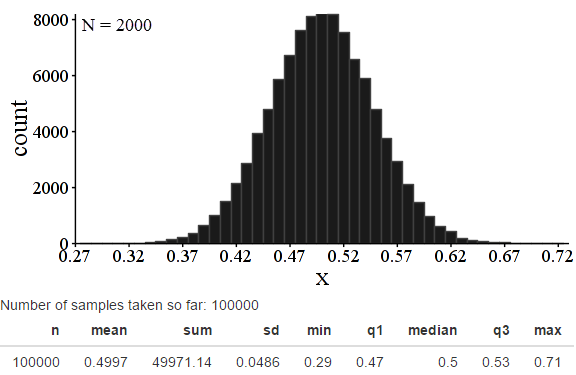Hmm. As suspected, my standard deviation dropped a bit, to 0.0486. But it’s not a lot. In practical usage (for hypothesis tests or confidence intervals), almost certainly not enough to matter much. So a population 20 times the sample seems okay.

Next, a population of 1500.Okay! Pretty much the same. Tiny bit smaller, but still reasonable. A population 15 times the sample checks out reasonably. Time to try a population of just 1000.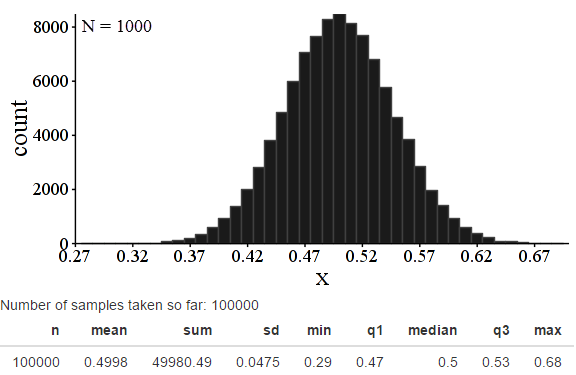Hmm. Hmmmmmmmmmmmmmm. Our standard deviation has dropped by a full 5% at this point from the original (and theoretical) value. Enough to matter in practice? Maaaayyyybe not, but definitely edging up on problematic. This is the absolute border of the 10% rule, note: the population is exactly 10 times the size of the sample.

Let’s keep going. Keep an eye on the “N = ???” at the upper right hand corner of these images to see the population size. Remember, sample size is 100 in every case.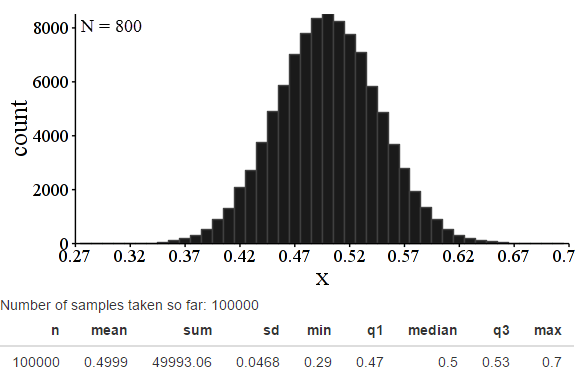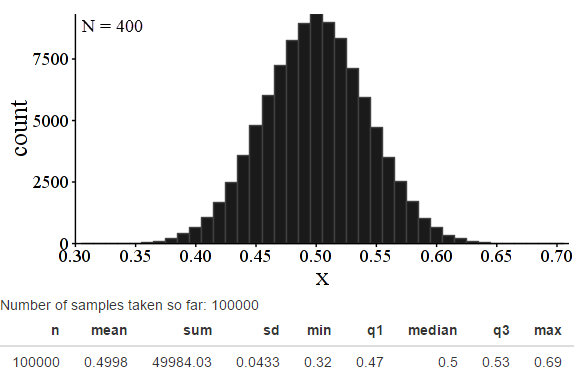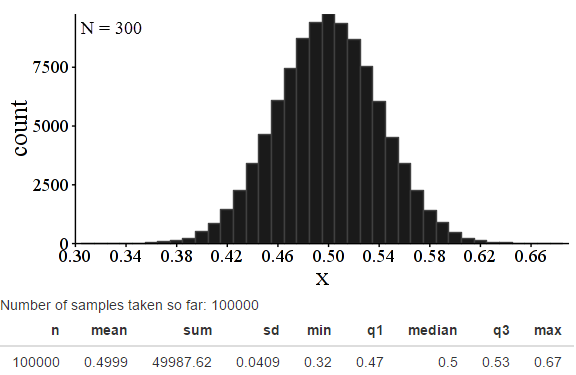Certainly, things have gone pretty badly by N = 200 – a population twice the sample size. And it’s definitely reasonable to think that the first arguably acceptable value is N=1000, when the population is 10 times the sample. But I also think you could reasonably argue that for particularly sensitive needs, even N = 2000 is too far below the theoretical value.

And that is why we need to really make clear – it is JUST a rule of thumb!

I haven’t figured out a way to investigate this with students yet, or if it’s even worth it; they mostly agree with me that N > 10n is a reasonable rule of thumb as is. But it’s pretty cool to have visual evidence of it for myself.

## 5 thoughts on “Using simulation to understand a statistical rule of thumb”

1.Khurram Siddiqui says:

Hi David ,

Your this post seems to me answer of why 10% sampling of population is rule of thumb. I am trying to understand it , can you please describe me the size of population and samples?

“I started by using the CPM e-Tool at http://shiny.mtbos.org/propsamples to generate 100,000 samples of size 100″, what is actual population and sample size in each iterative?

As you mentioned “but now I used a population of size 2000” , population of size 2000 , normally we use sample of population size N ? i am lost here in your each observation where you are validating 10% using population rather samples data

2.DavidGriswoldHH says:

Hi Khurram,

Sorry for the confusion!

The first time I ran the simulation, the first graph, uses samples of size n = 100 from a a theoretical “infiinte” population. That is, N = infinity. This is the equivalent of drawing with replacement and is the default setting with the propsamples applet (now available at http://stats.cpm.org/propsamples ) . This is the the theoretical model we base all proportion inference on.

In the second graph, I disabled the “draw with replacement” checkbox and used a sample size of N=2000, but still have a sample size of n=100. So each sample is only 5% of the population; therefore the “10% condition” is satisfied, and the graph looks pretty similar to the theoretical one.

The rest of the graphs keep sample size of n=100 but decrease the population until N=200. As the population size decreases (relative to the sample size) the spread of the sampling distribution decreases too, making the theoretical model less and less accurate.

Since doing this simulation, I’ve learned some more of the theory behind all of this: there is a corrective factor you can use called the FPC that corrects for situations like this, but for some reason the AP exam prefers to use the 10% condition.

3.Khurram Siddiqui says:

I think i cant play with this shiny apps , unable to reproduce what you have already produced 🙁

4.Khurram Siddiqui says:

thanks for your detail David 🙂